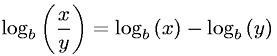Equations > Algebra > Logarithms > Difference of Logarithms Property

### Difference of Logarithms PropertyLatex Code:

MathML Code:

 ${\mathrm{log}}_{b}\left(\fracxy\right)={\mathrm{log}}_{b}\left(x\right)-{\mathrm{log}}_{b}\left(y\right)$

MathType 5.0: